Anzeige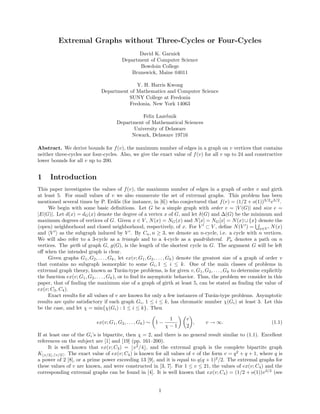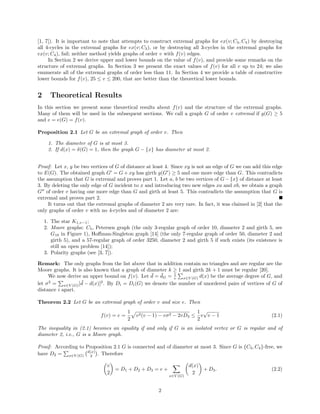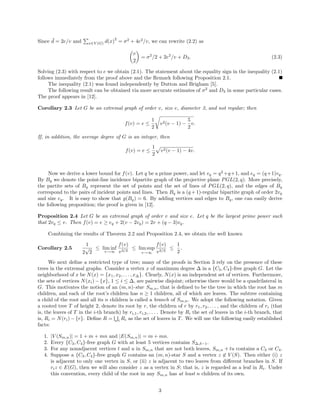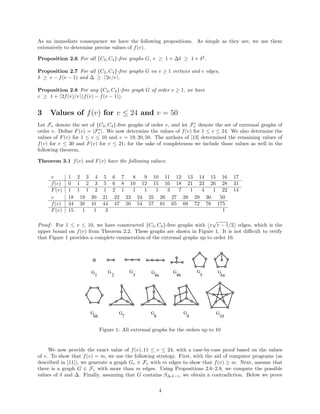Anzeige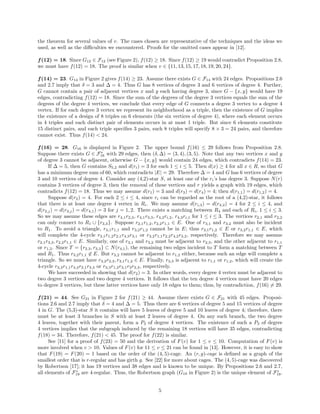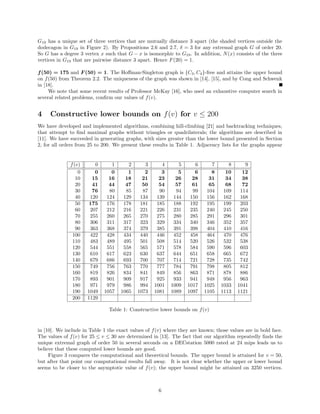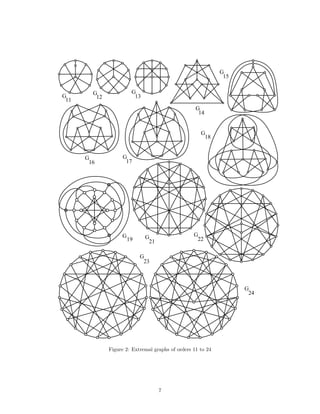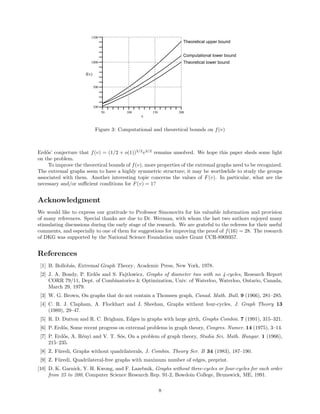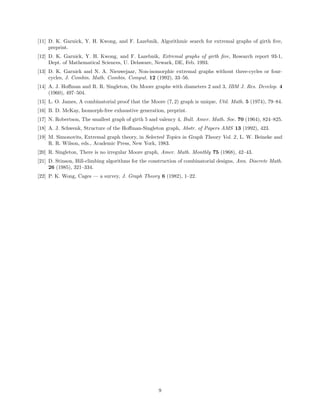Nächste SlideShare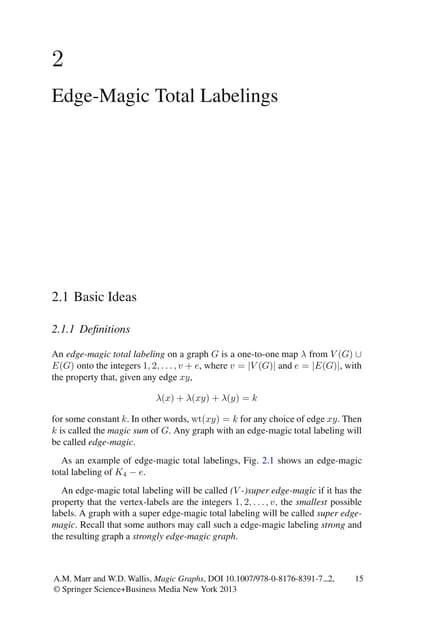Magic graphs
1 von 9
Anzeige

### 10.1.1.92.3502

1. Extremal Graphs without Three-Cycles or Four-Cycles David K. Garnick Department of Computer Science Bowdoin College Brunswick, Maine 04011 Y. H. Harris Kwong Department of Mathematics and Computer Science SUNY College at Fredonia Fredonia, New York 14063 Felix Lazebnik Department of Mathematical Sciences University of Delaware Newark, Delaware 19716 Abstract. We derive bounds for f (v), the maximum number of edges in a graph on v vertices that contains neither three-cycles nor four-cycles. Also, we give the exact value of f (v) for all v up to 24 and constructive lower bounds for all v up to 200. 1 Introduction This paper investigates the values of f (v), the maximum number of edges in a graph of order v and girth at least 5. For small values of v we also enumerate the set of extremal graphs. This problem has been mentioned several times by P. Erd˝s (for instance, in ) who conjectured that f (v) = (1/2 + o(1))3/2 v 3/2 . o We begin with some basic deﬁnitions. Let G be a simple graph with order v = |V (G)| and size e = |E(G)|. Let d(x) = dG (x) denote the degree of a vertex x of G, and let δ(G) and ∆(G) be the minimum and maximum degrees of vertices of G. Given x ∈ V , N (x) = NG (x) and N [x] = NG [x] = N (x) ∪ {x} denote the (open) neighborhood and closed neighborhood, respectively, of x. For V ⊂ V , deﬁne N (V ) = x∈V N (x) and V as the subgraph induced by V . By Cn , n ≥ 3, we denote an n-cycle, i.e. a cycle with n vertices. We will also refer to a 3-cycle as a triangle and to a 4-cycle as a quadrilateral. Pn denotes a path on n vertices. The girth of graph G, g(G), is the length of the shortest cycle in G. The argument G will be left oﬀ when the intended graph is clear. Given graphs G1 , G2 , . . . , Gk , let ex(v; G1 , G2 , . . . , Gk ) denote the greatest size of a graph of order v that contains no subgraph isomorphic to some Gi , 1 ≤ i ≤ k. One of the main classes of problems in extremal graph theory, known as Tur´n-type problems, is for given v, G1 , G2 , . . . , Gk to determine explicitly a the function ex(v; G1 , G2 , . . . , Gk ), or to ﬁnd its asymptotic behavior. Thus, the problem we consider in this paper, that of ﬁnding the maximum size of a graph of girth at least 5, can be stated as ﬁnding the value of ex(v; C3 , C4 ). Exact results for all values of v are known for only a few instances of Tur´n-type problems. Asymptotic a results are quite satisfactory if each graph Gi , 1 ≤ i ≤ k, has chromatic number χ(Gi ) at least 3. Let this be the case, and let χ = min{χ(Gi ) : 1 ≤ i ≤ k}. Then 1 v ex(v; G1 , G2 , . . . , Gk ) ∼ 1− , v → ∞. (1.1) χ−1 2 If at least one of the Gi ’s is bipartite, then χ = 2, and there is no general result similar to (1.1). Excellent references on the subject are  and  (pp. 161–200). It is well known that ex(v; C3 ) = v 2 /4 , and the extremal graph is the complete bipartite graph K v/2 , v/2 . The exact value of ex(v; C4 ) is known for all values of v of the form v = q 2 + q + 1, where q is a power of 2 , or a prime power exceeding 13 , and it is equal to q(q + 1)2 /2. The extremal graphs for these values of v are known, and were constructed in [3, 7]. For 1 ≤ v ≤ 21, the values of ex(v; C4 ) and the corresponding extremal graphs can be found in . It is well known that ex(v; C4 ) = (1/2 + o(1))v 3/2 (see 1
2. [1, 7]). It is important to note that attempts to construct extremal graphs for ex(v; C3 , C4 ) by destroying all 4-cycles in the extremal graphs for ex(v; C3 ), or by destroying all 3-cycles in the extremal graphs for ex(v; C4 ), fail; neither method yields graphs of order v with f (v) edges. In Section 2 we derive upper and lower bounds on the value of f (v), and provide some remarks on the structure of extremal graphs. In Section 3 we present the exact values of f (v) for all v up to 24; we also enumerate all of the extremal graphs of order less than 11. In Section 4 we provide a table of constructive lower bounds for f (v), 25 ≤ v ≤ 200, that are better than the theoretical lower bounds. 2 Theoretical Results In this section we present some theoretical results about f (v) and the structure of the extremal graphs. Many of them will be used in the subsequent sections. We call a graph G of order v extremal if g(G) ≥ 5 and e = e(G) = f (v). Proposition 2.1 Let G be an extremal graph of order v. Then 1. The diameter of G is at most 3. 2. If d(x) = δ(G) = 1, then the graph G − {x} has diameter at most 2. Proof: Let x, y be two vertices of G of distance at least 4. Since xy is not an edge of G we can add this edge to E(G). The obtained graph G = G + xy has girth g(G ) ≥ 5 and one more edge than G. This contradicts the assumption that G is extremal and proves part 1. Let a, b be two vertices of G − {x} of distance at least 3. By deleting the only edge of G incident to x and introducing two new edges xa and xb, we obtain a graph G of order v having one more edge than G and girth at least 5. This contradicts the assumption that G is extremal and proves part 2. It turns out that the extremal graphs of diameter 2 are very rare. In fact, it was claimed in  that the only graphs of order v with no 4-cycles and of diameter 2 are: 1. The star K1,v−1 ; 2. Moore graphs: C5 , Petersen graph (the only 3-regular graph of order 10, diameter 2 and girth 5, see G10 in Figure 1), Hoﬀman-Singleton graph  (the only 7-regular graph of order 50, diameter 2 and girth 5), and a 57-regular graph of order 3250, diameter 2 and girth 5 if such exists (its existence is still an open problem ); 3. Polarity graphs (see [3, 7]). Remark: The only graphs from the list above that in addition contain no triangles and are regular are the Moore graphs. It is also known that a graph of diameter k ≥ 1 and girth 2k + 1 must be regular . ¯ ¯ 1 We now derive an upper bound on f (v). Let d = dG = v x∈V (G) d(x) be the average degree of G, and ¯ let σ 2 = x∈V (G) [d − d(x)]2 . By Di = Di (G) we denote the number of unordered pairs of vertices of G of distance i apart. Theorem 2.2 Let G be an extremal graph of order v and size e. Then 1 1 √ f (v) = e = v 2 (v − 1) − vσ 2 − 2vD3 ≤ v v−1 (2.1) 2 2 The inequality in (2.1) becomes an equality if and only if G is an isolated vertex or G is regular and of diameter 2, i.e., G is a Moore graph. Proof: According to Proposition 2.1 G is connected and of diameter at most 3. Since G is {C3 , C4 }-free, we have D2 = x∈V (G) d(x) . Therefore 2 v d(x) = D1 + D2 + D3 = e + + D3 . (2.2) 2 2 x∈V (G) 2
3. ¯ Since d = 2e/v and 2 d(x) = σ 2 + 4e2 /v, we can rewrite (2.2) as x∈V (G) v = σ 2 /2 + 2e2 /v + D3 . (2.3) 2 Solving (2.3) with respect to e we obtain (2.1). The statement about the equality sign in the inequality (2.1) follows immediately from the proof above and the Remark following Proposition 2.1. The inequality (2.1) was found independently by Dutton and Brigham . The following result can be obtained via more accurate estimates of σ 2 and D3 in some particular cases. The proof appears in . Corollary 2.3 Let G be an extremal graph of order v, size e, diameter 3, and not regular; then 1 5 f (v) = e ≤ v 2 (v − 1) − v. 2 2 If, in addition, the average degree of G is an integer, then 1 f (v) = e ≤ v 2 (v − 1) − 4v. 2 Now we derive a lower bound for f (v). Let q be a prime power, and let vq = q 2 +q +1, and eq = (q +1)vq . By Bq we denote the point-line incidence bipartite graph of the projective plane P GL(2, q). More precisely, the partite sets of Bq represent the set of points and the set of lines of P GL(2, q), and the edges of Bq correspond to the pairs of incident points and lines. Then Bq is a (q + 1)-regular bipartite graph of order 2vq and size eq . It is easy to show that g(Bq ) = 6. By adding vertices and edges to Bq , one can easily derive the following proposition; the proof is given in . Proposition 2.4 Let G be an extremal graph of order v and size e. Let q be the largest prime power such that 2vq ≤ v. Then f (v) = e ≥ eq + 2(v − 2vq ) = 2v + (q − 3)vq . Combining the results of Theorem 2.2 and Proposition 2.4, we obtain the well known 1 f (v) f (v) 1 Corollary 2.5 √ ≤ lim inf 3/2 ≤ lim sup 3/2 ≤ . 2 2 v→∞ v v→∞ v 2 We next deﬁne a restricted type of tree; many of the proofs in Section 3 rely on the presence of these trees in the extremal graphs. Consider a vertex x of maximum degree ∆ in a {C3 , C4 }-free graph G. Let the neighborhood of x be N (x) = {x1 , x2 , . . . , x∆ }. Clearly, N (x) is an independent set of vertices. Furthermore, the sets of vertices N (xi ) − {x}, 1 ≤ i ≤ ∆, are pairwise disjoint; otherwise there would be a quadrilateral in G. This motivates the notion of an (m, n)-star Sm,n , that is deﬁned to be the tree in which the root has m children, and each of the root’s children has n ≥ 1 children, all of which are leaves. The subtree containing a child of the root and all its n children is called a branch of Sm,n . We adopt the following notation. Given a rooted tree T of height 2, denote its root by r, the children of r by r1 , r2 , . . . , and the children of ri (that is, the leaves of T in the i-th branch) by ri,1 , ri,2 , . . . . Denote by Ri the set of leaves in the i-th branch, that is, Ri = N (ri ) − {r}. Deﬁne R = i Ri as the set of leaves in T . We will use the following easily established facts: 1. |V (Sm,n )| = 1 + m + mn and |E(Sm,n )| = m + mn. 2. Every {C3 , C4 }-free graph G with at least 5 vertices contains S∆,δ−1 . 3. For any nonadjacent vertices t and u in Sm,n that are not both leaves, Sm,n + tu contains a C3 or C4 . 4. Suppose a {C3 , C4 }-free graph G contains an (m, n)-star S and a vertex z ∈ V (S). Then either (i) z is adjacent to only one vertex in S, or (ii) z is adjacent to two leaves from diﬀerent branches in S. If ri z ∈ E(G), then we will also consider z as a vertex in S; that is, z is regarded as a leaf in Ri . Under this convention, every child of the root in any Sm,n has at least n children of its own. 3
4. As an immediate consequence we have the following propositions. As simple as they are, we use them extensively to determine precise values of f (v). Proposition 2.6 For all {C3 , C4 }-free graphs G, v ≥ 1 + ∆δ ≥ 1 + δ 2 . Proposition 2.7 For all {C3 , C4 }-free graphs G on v ≥ 1 vertices and e edges, δ ≥ e − f (v − 1) and ∆ ≥ 2e/v . Proposition 2.8 For any {C3 , C4 }-free graph G of order v ≥ 1, we have v ≥ 1 + 2f (v)/v (f (v) − f (v − 1)). 3 Values of f (v) for v ≤ 24 and v = 50 ∗ Let Fv denote the set of {C3 , C4 }-free graphs of order v, and let Fv denote the set of extremal graphs of ∗ order v. Deﬁne F (v) = |Fv |. We now determine the values of f (v) for 1 ≤ v ≤ 24. We also determine the values of F (v) for 1 ≤ v ≤ 10 and v = 19, 20, 50. The authors of  determined the remaining values of f (v) for v ≤ 30 and F (v) for v ≤ 21; for the sake of completeness we include those values as well in the following theorem. Theorem 3.1 f (v) and F (v) have the following values: v 1 2 3 4 5 6 7 8 9 10 11 12 13 14 15 16 17 f (v) 0 1 2 3 5 6 8 10 12 15 16 18 21 23 26 28 31 F (v) 1 1 1 2 1 2 1 1 1 1 3 7 1 4 1 22 14 v 18 19 20 21 22 23 24 25 26 27 28 29 30 50 f (v) 34 38 41 44 47 50 54 57 61 65 68 72 76 175 F (v) 15 1 1 3 1 √ Proof: For 1 ≤ v ≤ 10, we have constructed {C3 , C4 }-free graphs with v v − 1/2 edges, which is the upper bound on f (v) from Theorem 2.2. These graphs are shown in Figure 1. It is not diﬃcult to verify that Figure 1 provides a complete enumeration of the extremal graphs up to order 10. G G G G G G G 1 2 3 4a 4b 5 6a G G G G G 6b 7 8 9 10 Figure 1: All extremal graphs for the orders up to 10 We now provide the exact value of f (v), 11 ≤ v ≤ 24, with a case-by-case proof based on the values of v. To show that f (v) = m, we use the following strategy. First, with the aid of computer programs (as described in ), we generate a graph Gv ∈ Fv with m edges to show that f (v) ≥ m. Next, assume that there is a graph G ∈ Fv with more than m edges. Using Propositions 2.6–2.8, we compute the possible values of δ and ∆. Finally, assuming that G contains S∆,δ−1 , we obtain a contradiction. Below we prove 4